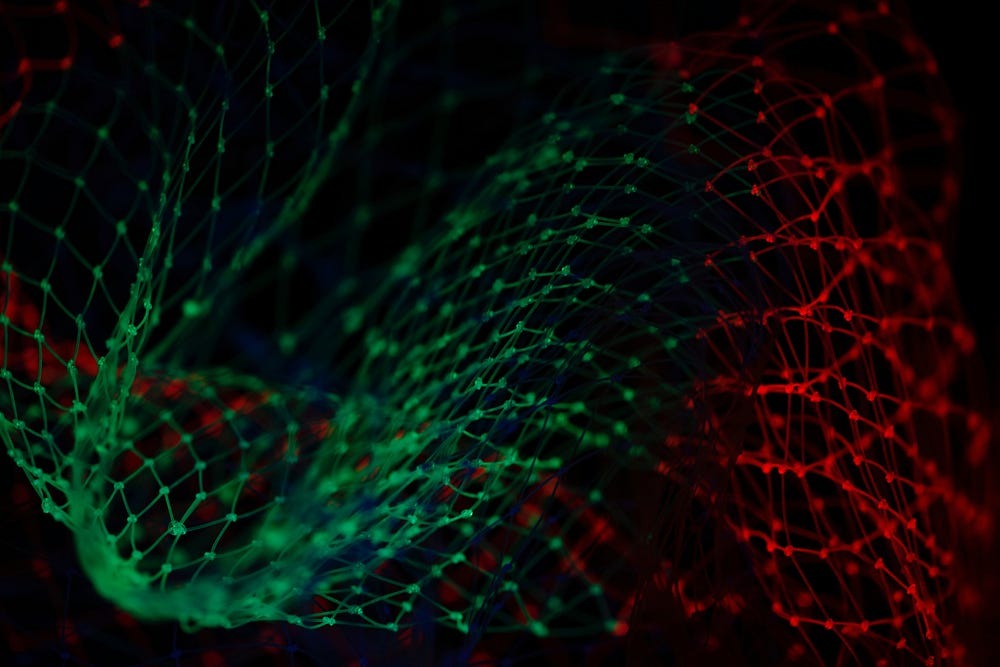# ResNets, DenseNets & UNets

Source: Deep Learning on Medium# “ResNets”

The most important question is how to train the deep convolutional networks? And quest to answer this question led to the invention of the ResNets. As per the traditional approach to train deeper convolutional neural networks, we increase the number of layers.

And as per our knowledge on convolutional neural networks, the below should happen — With the increase in the layers, the network should have low training error. But this was what didn’t happen!

• The deeper network is represented in “red,” and the shallow network is described in “yellow.”
• We can easily observe that the deep neural network has more training errors, which we didn’t expect.

The confusion led Kaiming He and other researchers to publish their research. Let us understand the idea.

## Deep Residual Learning

As per the above plain neural net, the output H(x) is the combined effect of 2 weight layers and then two non-linear ReLu layers on the input. If we could turn this plain net into some deeper net that sustains the impact of the shallow net with added layers at the same time, then after the operation, the deeper network would at least retain the effect of the process on the shallow network. The same approach led to the phenomenon of the ResNets

• In ResNet, we add a layer which is the addition of the `F(x)` and `x` i.e. identity function.
• This helps in solving the problem of dissolving gradient by allowing an alternative path for the gradient to flow through. Also, they use identity function, which allows a higher layer to perform as good as a lower layer, and not worse at least.
• Now, after every two-weight layers and 1 Relu operation, we add to the output of the neural process, the identity. Thus, it would retain at least the effect of 20 layers on a deep 56 layers network in the situation when we start adding the impact of the 20 layer operation on the added 36 layers to the network.
• When the back-propagation is done through identity function, the gradient will be multiplied only by 1. This preserves the input and avoids any loss in the information.